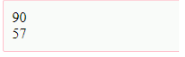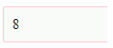# How to convert Char to Int in Java?

Last updated on Sep 19,2019 684 Views22 / 29 Blog from Java Programs

In Java, the value contained in one data type can be converted to another data type based on conversion requirements, the same holds true for char to int conversion. Converting a char to an int is equivalent to finding the ASCII value of the given character. In this article on “Convert Char to Int in Java”, we will learn how to convert a char to int with the help of examples.

## What are Data types?

Data types are the storage containers that store the value of a particular type like integers, chars, floating values, Boolean values, etc. A data type specifies the type of value a variable has and the type of relational, mathematical or logical operations that can be applied to it without causing an error.

The choice of data types for a particular variable depends on multiple factors. In most cases, the decision is intuitive. For example,

• If you want to store a numeric value then declare the data type like int
• For floating storage declare data type as Float
• For storing text messages, declare data type as the string
• To store a single character, declare data type like char
• For storing binary values, either True or False, declare the data type as Boolean

## Java char to int: Getting ASCII value

Filename: CharToIntExample.java

Code:

```public static void main(String args[]){
char c='Z';
char c1='9';
int a=c;
int b=c1;
System.out.println(a);
System.out.println(b);
}}
```

Compile by : javac CharToIntExample.java
Run by : java CharToIntExample

Output## Java char to int Example: Character.getNumericValue()

Filename: CharToIntExample1.java

Code:

```public class CharToIntExample1{
public static void main(String args[]){
char c='9';
int a=Character.getNumericValue(c);
System.out.println(a);
}}
```

Compile by: javac CharToIntExample1.java
Run by: java CharToIntExample1

Output:Java char to int Example: String.valueOf() :

Filename: CharToIntExample2.java

Code:

```public class CharToIntExample2{
public static void main(String args[]){
char c='8';
int a=Integer.parseInt(String.valueOf(c));
System.out.println(a);
}}
```

Compile by: javac CharToIntExample2.java

Run by: java CharToIntExample2

Output: